Next: 8.7 Programming Assignments Up: 8. Undirected Graphs Previous: 8.5 To Probe Further

# 8.6 Problems

1.
Consider an undirected graph G=(V, E), with n vertices. Show how a one dimensional array of length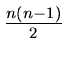can be used to represent G.
2.
Give an algorithm that determines whether or not a given undirected graph G = (V, E) contains a cycle. The algorithm should run in O(|V|) time independent of |E|.
3.
Design an algorithm to enumerate all simple cycles of a graph. How many such cycles can there be? What is the time complexity of the algorithm?
4.
Let (u, v) be a minimum-weight edge in a graph G. Show that (u, v) belongs to some minimum spanning tree of G.
5.
Let e be maximum-weight edge on some cycle of G = (V, E). Prove that there is a minimum spanning tree of G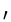= (V, E - {e}) that is also a minimum spanning tree of G.
6.
For an undirected graph G with n vertices and e edges, show that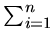di = 2e where di is the degree of vertex i (number of edges incident on vertex i).
7.
The diameter of a tree T = (V, E) is defined by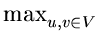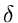(u, v) where(u, v) is the shortest-path distance from vertex u to vertex v. Give an efficient algorithm to compute the diameter of a tree, and analyze the running time of the algorithm.
8.
An Eulerian walk in an undirected graph is a path that starts and ends at the same vertex, traversing every edge in the graph exactly once. Prove that in order for such a path to exist, all nodes must have even degree.
9.
Two binary trees T1 and T2 are said to be isomorphic if T1 can be transformed into T2 by swapping left and right children of (some of the) nodes in T1. Design an efficient algorithm to decide id two given trees are isomorphic. What is the time complexity of your algorithm?Next: 8.7 Programming Assignments Up: 8. Undirected Graphs Previous: 8.5 To Probe Further
eEL,CSA_Dept,IISc,Bangalore